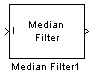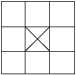# Median Filter

Perform 2-D median filtering

## Library

Filtering and Analysis & Enhancement

`visionanalysis`

`visionfilter`

•## Description

The Median Filter block replaces the central value of an M-by-N neighborhood with its median value. If the neighborhood has a center element, the block places the median value there, as illustrated in the following figure.The block has a bias toward the upper-left corner when the neighborhood does not have an exact center. See the median value placement in the following figure.The block pads the edge of the input image, which sometimes causes the pixels within [M/2 N/2] of the edges to appear distorted. The median value is less sensitive than the mean to extreme values. As a result, the Median Filter block can remove salt-and-pepper noise from an image without significantly reducing the sharpness of the image.

PortInput/OutputSupported Data TypesComplex Values Supported

I

Matrix of intensity values

• Double-precision floating point

• Single-precision floating point

• Fixed point

• Boolean

• 8-, 16-, 32-bit signed integer

• 8-, 16-, 32-bit unsigned integer

No

Val

Scalar value that represents the constant pad value

Same as I port

No

Output

Matrix of intensity values

Same as I port

No

If the data type of the input signal is floating point, the output has the same data type. The data types of the signals input to the I and Val ports must be the same.

### Fixed-Point Data Types

The information in this section is applicable only when the dimensions of the neighborhood are even.

For fixed-point inputs, you can specify accumulator and output data types as discussed in Parameters. Not all these fixed-point parameters apply to all types of fixed-point inputs. The following table shows the output and accumulator data type used for each fixed-point input.

Fixed-Point Input

Output Data Type

Accumulator Data Type

Even `M`

X

X

Odd `M`

X

Odd `M` and complex

X

X

Even `M` and complex

X

X

When `M` is even, fixed-point signals use the accumulator and output data types. The accumulator data type store the result of the sum performed while calculating the average of the two central rows of the input matrix. The output data type stores the total result of the average.

Complex fixed-point inputs use the accumulator parameters. The calculation for the sum of the squares of the real and imaginary parts of the input occur, before sorting input elements. The accumulator data type stores the result of the sum of the squares.

## Parameters

Neighborhood size

Specify the size of the neighborhood over which the block computes the median.

• Enter a scalar value that represents the number of rows and columns in a square matrix.

• Enter a vector that represents the number of rows and columns in a rectangular matrix.

Output size

This parameter controls the size of the output matrix.

• If you choose `Same as input port I`, the output has the same dimensions as the input to port `I`. The Padding options parameter appears in the dialog box. Use the Padding options parameter to specify how to pad the boundary of your input matrix.

• If you select `Valid`, the block only computes the median where the neighborhood fits entirely within the input image, with no need for padding. The dimensions of the output image are, ```output rows = input rows - neighborhood rows + 1```,
and
```output columns = input columns - neighborhood columns + 1```.

• Select `Constant` to pad your matrix with a constant value. The Pad value source parameter appears in the dialog box

• Select `Replicate` to pad your input matrix by repeating its border values.

• Select `Symmetric` to pad your input matrix with its mirror image.

• Select `Circular` to pad your input matrix using a circular repetition of its elements. This parameter appears if, for the Output size parameter, you select ```Same as input port I```.

Use this parameter to specify how to define your constant boundary value.

• Select `Specify via dialog` to enter your value in the block parameters dialog box. The Pad value parameter appears in the dialog box.

• Select `Input port` to specify your constant value using the `Val` port. This parameter appears if, for the Padding options parameter, you select `Constant`.

Enter the constant value with which to pad your matrix. This parameter appears if, for the Pad value source parameter, you select `Specify via dialog`. Tunable.

Rounding mode

Select the rounding mode for fixed-point operations.

Overflow mode

Select the overflow mode for fixed-point operations.

### Note

Only certain cases require the use of the accumulator and output parameters. Refer to Fixed-Point Data Types for more information.

Accumulator

Use this parameter to specify the accumulator word and fraction lengths resulting from a complex-complex multiplication in the block:

• When you select `Same as input`, these characteristics match the related input to the block.

• When you select `Binary point scaling`, you can enter the word length and the fraction length of the accumulator, in bits.

• When you select `Slope and bias scaling`, you can enter the word length, in bits, and the slope of the accumulator. This block requires power-of-two slope and a bias of 0.

Output

Choose how to specify the output word length and fraction length:

• When you select `Same as input`, these characteristics match the related input to the block.

• When you select `Binary point scaling`, you can enter the word length and the fraction length of the output, in bits.

• When you select `Slope and bias scaling`, you can enter the word length, in bits, and the slope of the output. This block requires power-of-two slope and a bias of 0.

Lock data type settings against change by the fixed-point tools

Select this parameter to prevent the fixed-point tools from overriding the data types you specify on the block mask. For more information, see `fxptdlg`, a reference page on the Fixed-Point Tool in the Simulink® documentation.

## References

 Gonzales, Rafael C. and Richard E. Woods. Digital Image Processing. 2nd ed. Englewood Cliffs, NJ: Prentice-Hall, 2002.

 2-D Convolution Computer Vision Toolbox™ 2-D FIR Filter Computer Vision Toolbox `medfilt2` Image Processing Toolbox™